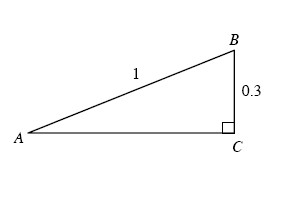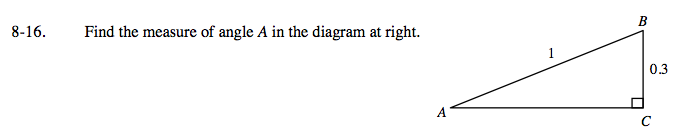Home > A2C > Chapter 8 > Lesson 8.1.2 > Problem8-16

8-16.

Find the measure of angle A in the diagram below. Homework Help ✎The length 0.3 is opposite angle A and the length 1 is the hypotenuse.
Which trigonometric relationship works with opposite and hypotenuse?

$\text{sin}A=\frac{0.3}{1}$

A = sin¹(0.3) ≈ 17.46°